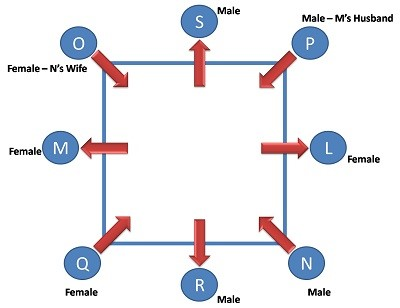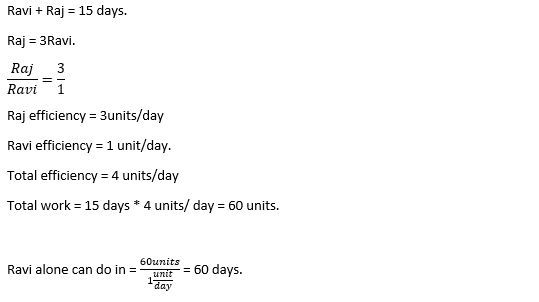# Infosys Mathematical Ability Previous Year Questions

28 January 2023

Infosys Previous Year Questions

Check below videos for previous year questions:

Reasoning Ability

Mathematical Ability

Verbal Ability

Psuedocode and Coding (For SP and DSE Roles)

Puzzle solving

Infosys Mathematical Ability Questions

Q1) If (RT)^3 = FELT, then what is the value of R+E+L+T? (All the letters take unique value except 0)

A. 22

B. 25

C. 23

D. 20

E. 21

Expnanation

RT3 = FELT.

As per the condition, when the unit digit in RT is cubed. The resultant number also should end as T.

So, the possibilities when a digit is cubed and gives the same digit are 4, 5, 6, 9.

143= 2744. Since every alphabet takes unique value. LT = 44, so can’t be possible.

153= 3375. Since every alphabet takes unique value. FE = 33, so can’t be possible.

163 = 4096. Since no letter takes 0. This can’t be possible number.

193= 6859. This number fulfils the condition perfectly.

So, FELT = 6859.

R + E + L + T = 1 + 8 + 5 + 9 = 23.

Q2) Directions: L,M,N,O,P,Q,R and S are sitting around a square table in such a way that four of them sit at the four corners of the square while four sits in the middle of each side. The ones who sit at the corners face the centre of the square while those who sit in the middle of each side face outside. Two females sit at the middle of the sides and two sit at the corners.

-  L sits second to the left of R.

-  R sits in the middle of one of the sides.

-  N sits fourth to the right of his wife and his wife is not an immediate  neighbor of L or R.

-  M sits third to the right of her husband.

-  M does not sit at any of the corners.

-  Only O sits between M and S.

-  S is the husband of L.

P is a male.

Which of the following is true with respect to the given sitting arrangement?

A. No two males are immediate neighbors of each other.

B. R and S face each other in the sitting arrangement.

C. L sits in the middle of one of the sides of the square table.

D. Q is a male and sits diagonally opposite to P.

E. None of these

Explanation:
According the conditions, the arrangement as follows.Q3) Ravi and Raj can complete a work in 15 days if they work together . Raj is thrice as efficient as Ravi. If Ravi works alone how many days will he take to complete the same work?

A. 60 days

B. 20 days

C. 15 days

D. 40 days

Explanation:Q4) A can complete a work in 20 days , B completes the work in 30 days and C completes it in 60 days. In how many days will the work be completed if A is assisted by B and C in every 4th day?

A. 12

B. 15

C. 16

D. 18

Explanation:Q5) How many 3-digit numbers can be formed from the digits 2, 3, 4, 5, 6, 7 and 9 (without repetition), which are divisible by 5?

A. 10

B. 15

C. 5

D. 30

Explanation:

Since each desired number is divisible by 5, so we must have 5 at the unit place. So, there is 1 way of doing it.

The tens place can now be filled by any of the remaining 6 digits (2, 3, 4, 6, 7, 9). So, there are 6 ways of filling the tens place.

The hundreds place can now be filled by any of the remaining 5 digits. So, there are 5 ways of filling it.

number of3 digit numbers can be formed are = (5 x 6 x 1) = 30.

Q6) 20 years before, the age of Rony’s father was 12 times of Rony’s age. At present, his age is 2 times of Rony’s age. Find the present age of Rony.

A. 20 years

B. 24 years

C. 26 years

D. 22 years

Explanation:

The present age of Rony? is 22 years

Step-by-step explanation:

Let present age of the son =x

Present age of the father =2x

Now, according to question, we have to find

2x−20=12×(x−20)

2x−20=12x−240

10x=220

x=22

Present age of son =22 years

Present age of father =2×22=44 years.

Hence, we get the present age of Rony? as 22 years

Q7) Select the pair that is related on the same basis to the pair given in the question.

FIZU : HABI :: ?

A. MEVO : OOXA

B. CATI : EYRU

C. HEPO : FORI

D. LICA : NAEO

Explanation:

Logic between the numerical place values of the alphabets is

FIZU = 6    9    26 or 0     21

+2   -8       +2        -12

HABI = 8    1        2           9

The only option which has the same logic is Option D.

LICA =   12   9      3    1 or 21

+2   -8   +2    -12

NAEO = 14   1      5     15.

Q8) Which two signs should be interchanged to make the below equation mathematically correct?

4 + 8 ÷ 16 x 13 - 7 = 8

A. - and +

B. x and ÷

C. + and x

D. ÷ and -

Explanation:

When going with option verification, only when + and x are interchanged the equation can be satisfied.

4 x 8 ÷ 16 + 13 – 7 = 8.

Q9) If signs ‘+’ and ‘-’ and numbers 7 and 6 are used interchangeably, then which of the following equation would be correct?

A. 7 x 2 + 6 - 5 = 8

B. 5 + 7 x 2 -6 = 0

C. 7 -6 + 3 x 5 = 30

D. 6 x 7 + 5 -8 =20

Explanation:

When going with option verification, only interchanging in option B will satisfy the equation.

Option B - 5 + 7 x 2 -6 = 0, which is actually not correct.

After interchanging as per the question,

5 – 6 x 2 + 7 = 0.

Q10) If ‘+’ means ‘x’, ‘÷’ means ‘+’, ‘-’ means ‘÷’ and ‘x’ means ‘-’. Which of the following will be the value of the expression 32 ÷ 8 - 4 x 12 + 4 = ?

A. 12

B. 1/12

C. 40

D. -14

Explanation:

Given expression = 32 ÷ 8 - 4 x 12 + 4

Changing the operators as per the question, the final expression will be,

32 + 8 ÷ 4 – 12 x 4 = - 14.

Q11) If ‘+’ means ‘-’, ‘-’ means ‘÷’, ÷ ‘’ means ‘x’, and ‘x’ means ‘+’. Which of the following will be the value of the expression?

245 - 35 ÷ 3 + 14 x 36

A. 58

B. 45

C. 37

D. 43

Explanation:

Given expression = 245 - 35 ÷ 3 + 14 x 36

Changing the operators as per the question, the final expression will be,

245 ÷ 35 x 3 - 14 + 36 = 43.

Q12) In a class, Ram’s rank from top is 12 and Ramesh’s rank from bottom is 27. If they interchange their positions, Ram’s rank becomes 18th from the top. How many students are there in the class?

A. 42

B. 43

C. 45

D. 44

Explanation:

Given that,

12th is the rank of Ram from the top.

27th is the rank of Ramesh from the bottom.

That means,

There are 11 students are above Ram.

And 26 students are below Ramesh.

After they interchanged their positions,

Ram's rank became at 18th.

Then,

there will be 17 students above.

As Ram is now in Ramesh's position there are 26 students below Ram.

Therefore,

Total students = 17+26+1

Total students = 44.

Q13) Consider the number 57645361, if the digit at unit place is decreased by 1, the digit at tens ‘place is increased by 2, the digit at hundreds’ place is decreased by 1, the digit at thousands’ place is increased by 2 and so on, then after rearranging how many number appears twice?

A. 2

B. 3

C. 4

D. 1

Explanation:

Number is: 57645361

the digit at unit place is decreased by 1 -> 57645360

the digit at ten’s place is increased by 2 -> 57645380

the digit at hundreds’ place is decreased by 1 -> 57645280

the digit at thousands’ place is increased by 2 -> 57647280

and so on for remaining digits going with -1, +2, -1, +2 format gives the below numbers

57637280

57837280

56837280

76837280.

Since all the digits were applied with the given logic, at the end the final number obtained is 76837280. In which 7 and 2 appeared twice. So, 2 numbers appeared twice.

Q14) In a certain code language, ‘FAN’ is written as “21”, then how will “COME” be written in that code language?

A. 35

B. 34

C. 36

D. 37

Explanation:

The logic given for the word FAN is adding the alphabetical place values.

FAN – 6 1 14 = 6+1+14 = 21.

Applying the same logic,

COME – 3 15 13 5 = 3 + 15 + 13 + 5 = 36.

Q15) If ‘A’ means ‘subtraction’, ‘B’ means ‘division’, ‘C’ means ‘multiplication’, and ‘D’ means ‘addition’, then what will be the value of the following expression?

18 A 15 B 75 C 90 D 23

A. 18

B. 15

C. 23

D. 33

Explanation:

Applying the conditions from the data in the question changes the expression from 18 A 15 B 75 C 90 D 23 to 18 - 15 ÷ 75 x 90 + 23.

The answer for the expression, 18 - 15 ÷ 75 x 90 + 23. is 23.

Infosys Pattern and Syllabus

##